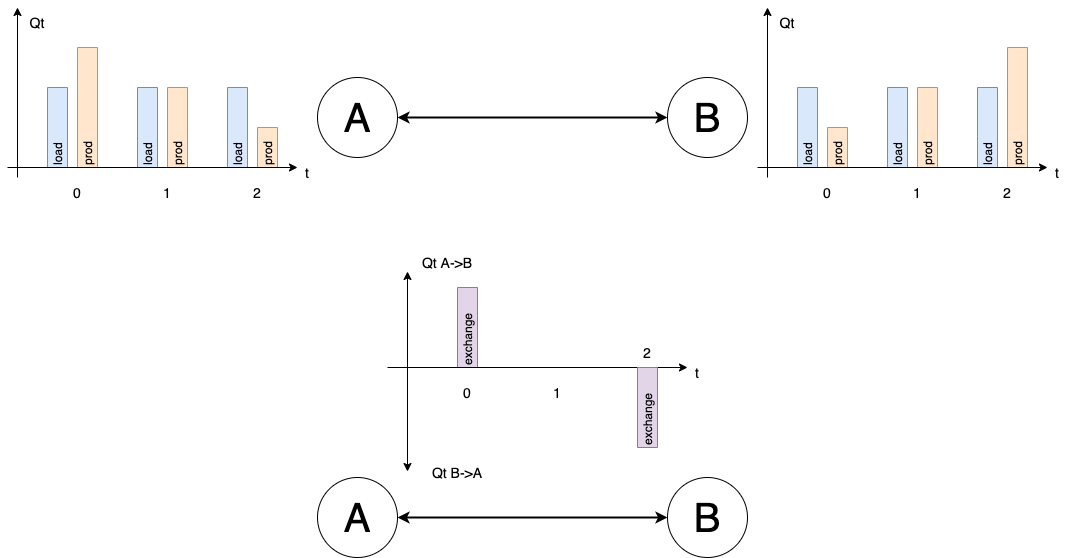# Get Started¶

Except where otherwise noted, this content is Copyright (c) 2020, RTE and licensed under a CC-BY-4.0 license.

Each kind of network has a needs of adequacy. On one side, some network nodes need to consume items such as watt, litter, package. And other side, some network nodes produce items. Applying adequacy on network, is tring to find the best available exchanges to avoid any lack at the best cost.

For example, a electric grid can have some nodes wich produce too more power and some nodes wich produce not enough power.In this case, at t=0, A produce 10 more and B need 10 more. Then nodes are well balanced. And at t=2, B produce 10 more and A need 10 more.

For this example, perform adequacy will done ten quantities exachanges from A to B, then zero and at the end 10 quantities from B to A.

Hadar compute adequacy from simple to complex network. For example, to compute above network, just few line need:

Firstly, install hadar : pip install hadar

import hadar as hd

study = hd.Study(horizon=3)\
.network()\
.node('a')\
.consumption(cost=10 ** 6, quantity=[20, 20, 20], name='load')\
.production(cost=10, quantity=[30, 20, 10], name='prod')\
.node('b')\
.consumption(cost=10 ** 6, quantity=[20, 20, 20], name='load')\
.production(cost=10, quantity=[10, 20, 30], name='prod')\
.link(src='a', dest='b', quantity=[10, 10, 10], cost=2)\
.link(src='b', dest='a', quantity=[10, 10, 10], cost=2)\
.build()

optimizer = hd.LPOptimizer()
res = optimizer.solve(study)


Then you can analyze by yourself result or use hadar aggragator and plotting

plot = hd.HTMLPlotting(agg=hd.ResultAnalyzer(study, res),
node_coord={'a': [2.33, 48.86], 'b': [4.38, 50.83]})

plot.network().node('a').stack()